## 20150728

### Astronomy in-class activity: planet-hunting

Astronomy 210 In-class activity 6 v.15.07.28, fall semester 2015
Cuesta College, San Luis Obispo, CA

Students find their assigned groups of three to four students, and work cooperatively on an in-class activity worksheet to determine where in the sky each naked-eye planet will be observed on a given date (here, September 2, 2015).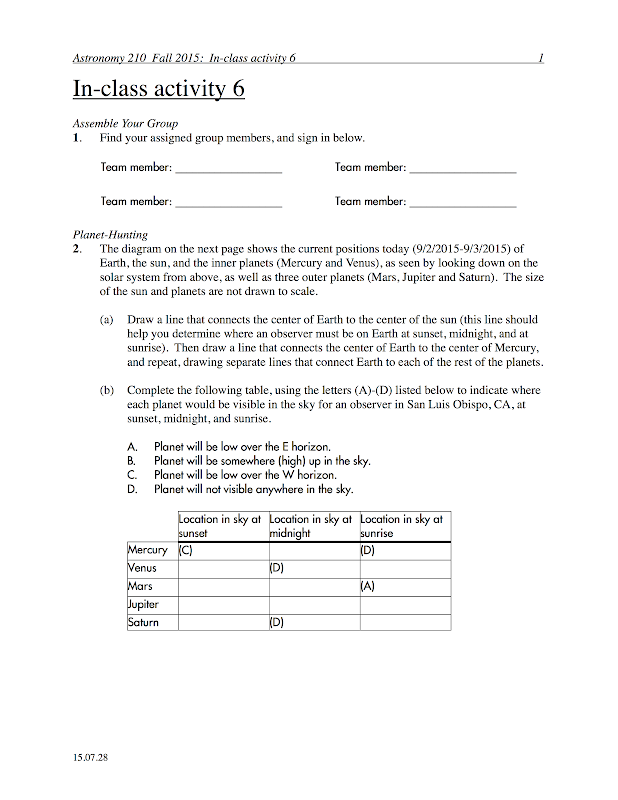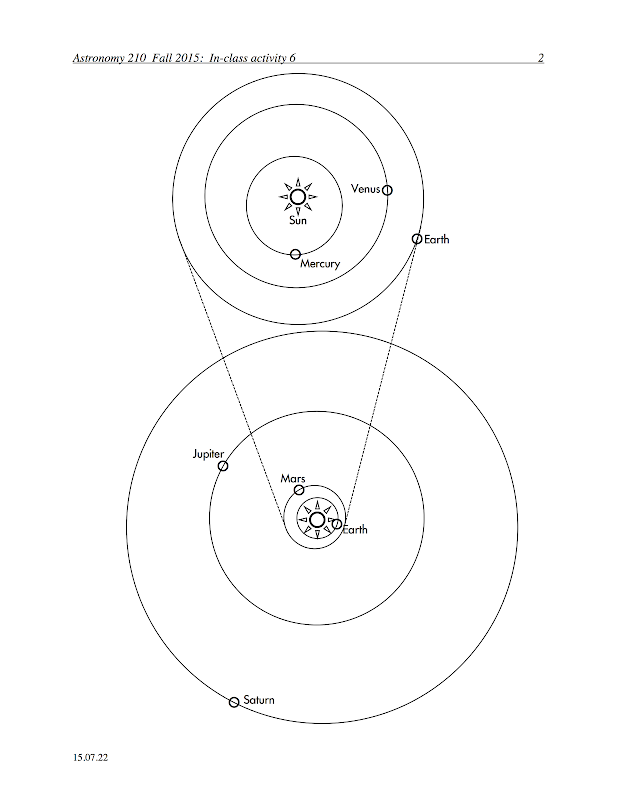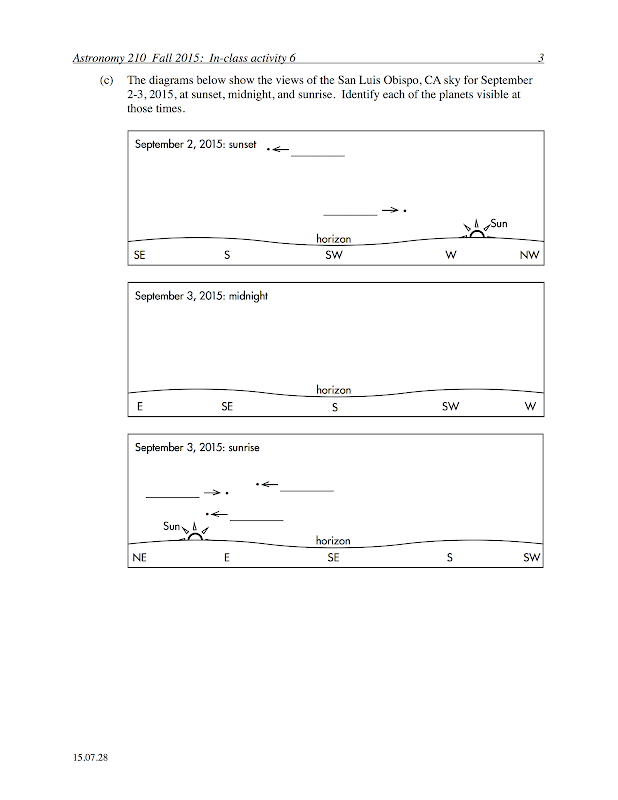Previous posts:

## 20150722

### Physics presentation: speed and velocityPlanes, planes, and more planes. Oh wait, that's just the same plane, isn't it? (Movie link: "Paths of Flight.")Let's introduce the different types of (one-dimensional) velocities used to describe motion along a line.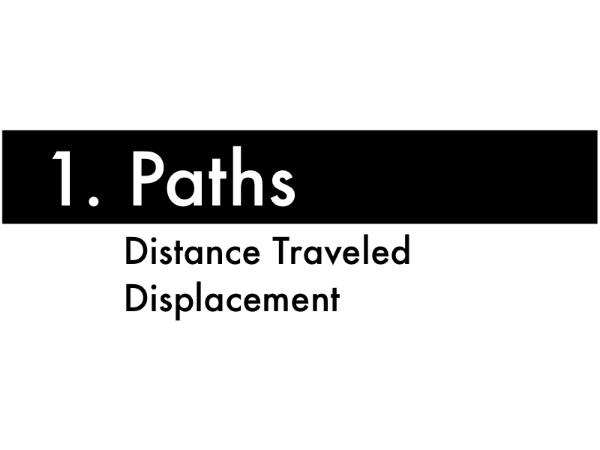We'll first need to define two different types of "paths," as somehow measured from an initial starting point to a final ending point.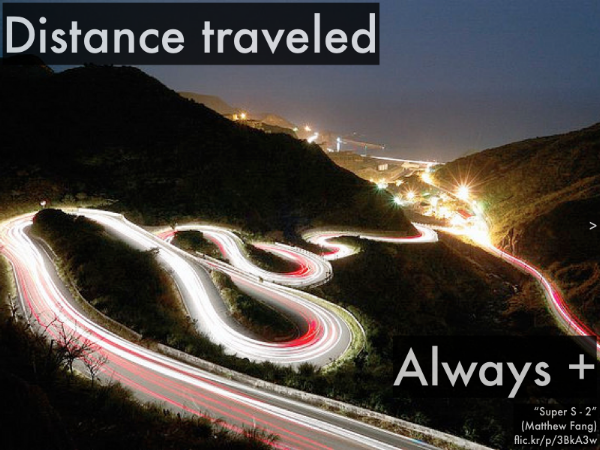Distance traveled is conventionally how far one would travel, regardless of direction, even going back-and-forth. This is always a positive quantity, as even moving backwards is counted as distance traveled.Displacement is the straight-line distance from the initial starting point, to the final ending point--"as the crow flies" (but not necessarily how the pigeon walks). This can be positive or negative, depending on the direction from the initial to the final location, for horizontal displacements we will use positive values to indicate displacements that point to the right, and negative values to indicate displacements that point to the left. This is always a straight line, regardless of the back-and-forth distance traveled while en route from the initial starting point to the final ending point.
In general, distance traveled will be __________ the magnitude of displacement.
(A) less than.
(B) equal to.
(C) greater.
(D) (More than one of the above choices.)
(E) (None of the above choices.)
(F) (Unsure/guessing/lost/help!)With these two types of paths defined, now let's consider speed, and as it turns out, two different types of velocity.Consider the contrail left by this airplane--definitely not straight-line travel. Estimating the distance along this curving path, and dividing that distance traveled by the time interval spent traveling along this path...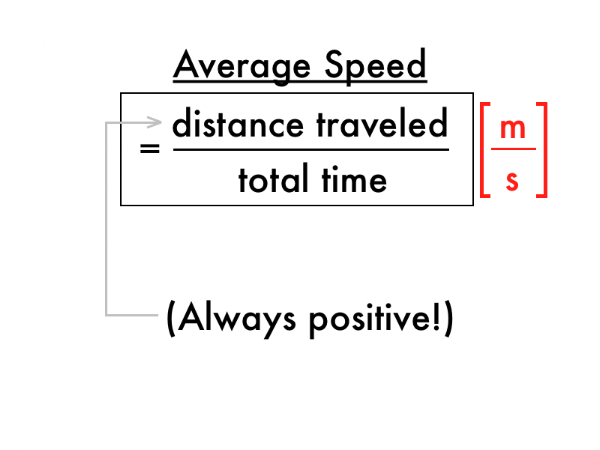...would result in the average speed of the aircraft, as it traveled along this path. Note that this is always a positive quantity.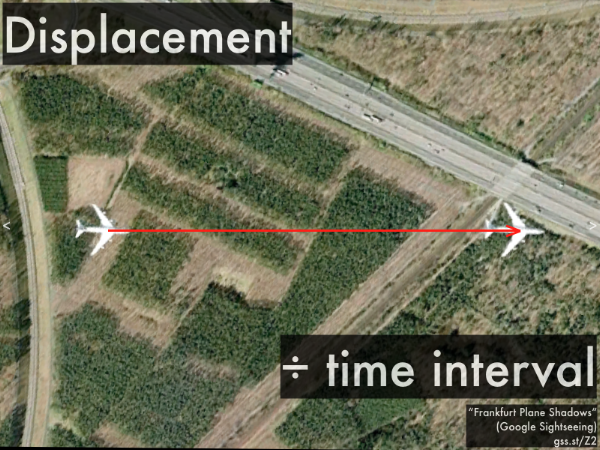This is an actual view from Google Maps (courtesy of Google Sightseeing). The same plane is shown in several different locations, as seen from a satellite overhead, as it was captured on consecutive vertical passes of the imaging camera. Knowing the displacement--the straight-line distance from the initial starting point to the final ending point--and dividing that by the time interval spent traveling along this displacement......would result in the average velocity of the aircraft, as it traveled along this displacement. Note that this can be either a positive or a negative quantity, depending on the direction of the displacement. (Some textbooks draw a horizontal "bar" over the "vx" to denote that it is an average quantity, which could be confused with a vector arrow--here we denote an average quantity by labeling it in the subscript, in order to avoid this confusion.)
In general, average speed will be __________ the magnitude of average velocity.
(A) less than.
(B) equal to.
(C) greater than.
(D) (More than one of the above choices.)
(E) (None of the above choices.)
(F) (Unsure/guessing/lost/help!)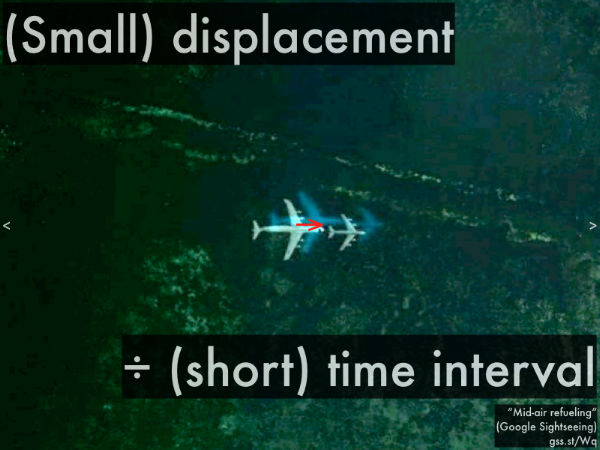Another actual view from Google Maps (again, courtesy of Google Sightseeing). Note the detailed monochrome image of these two planes, and the matching corresponding blurry color image for each plane. This is due to the satellite camera making two separate monochrome and color images on the same pass, but separated by a very brief time interval. In this case, taking the straight-line displacement between the initial starting point to the final ending point is correspondingly small--and dividing that by the brief time interval spent traveling along this displacement......would result in what would approach the instantaneous velocity of the aircraft, as it traveled along this small displacement during a brief time interval. (Note again that this can be either a positive or a negative quantity, depending on the direction of the displacement.) To properly make this an instantaneous velocity, we would do some calculus mumbo-jumbo where the limit of a vanishingly small time interval is taken in dividing into a correspondingly infinitesimal displacement.

So we have average speed, average velocity, and instantaneous velocity (although we often leave out "instantaneous" when referring to velocity, as that is implied, but strive to always explicitly leave in the "average" terminology).
In general, which of the following quantities could be negative?
(A) Average velocity.
(B) Average speed.
(C) (Instantaneous) velocity.
(D) (Instantaneous) speed.
(E) (More than one of the above choices.)
(F) (All of the above choices.)
(G) (None of the above choices.)
(H) (Unsure/guessing/lost/help!)What about (instantaneous) speed? How is it related to (instantaneous) velocity?
In general, (instantaneous) speed will be __________ the magnitude of (instantaneous) velocity.
(A) less than.
(B) equal to.
(C) greater than.
(D) (More than one of the above choices.)
(E) (None of the above choices.)
(F) (Unsure/guessing/lost/help!An odometer measures an object's:
(A) displacement.
(B) distance traveled.
(C) (instantaneous) velocity.
(D) (instantaneous) speed.
(E) (Unsure/guessing/lost/help!)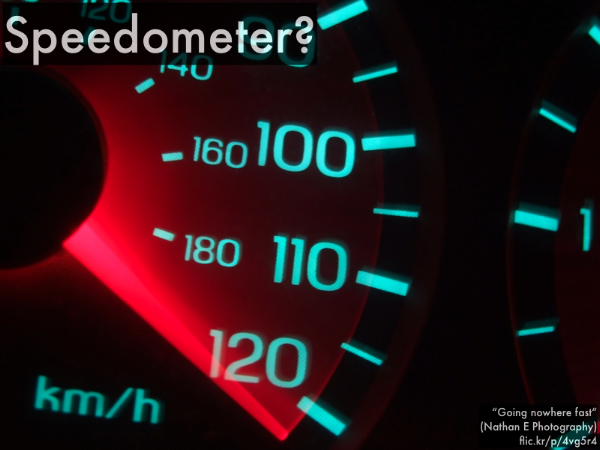And your car's speedometer--what quantity does that measure?
A speedometer measures an object's:
(A) displacement.
(B) distance traveled.
(C) (instantaneous) velocity.
(D) (instantaneous) speed.
(E) (Unsure/guessing/lost/help!)

## 20150719

### Physics presentation: forces and motionPreviously we introduced forces: how objects interact with each other, represented by vectors, which we introduced even earlier. Let's tie together forces, motion, and vector addition.Now we'll introduce Newton's first law and second law, which connect forces with motion. In order to determine whether Newton's first law or second law applies, we can either consider the motion of an object, or the net force on an object.First we'll analyze the motion of an object, in order to determine which Newton's law applies to it.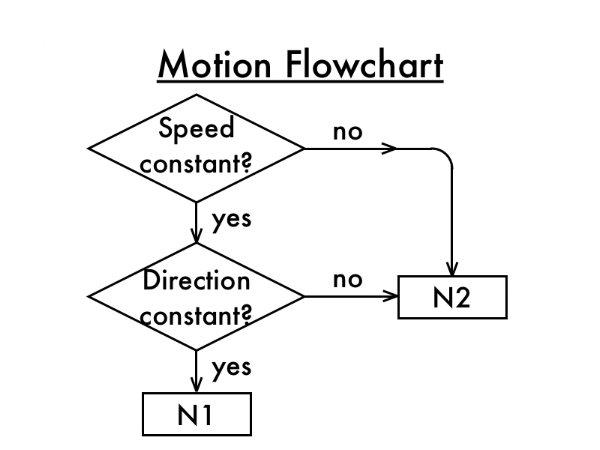This flowchart asks two questions to distinguish between Newton's first law and second law cases.

First, is the speed constant? If not, then Newton's second law applies. But if yes, speed is constant, then you can move on to the second question.

Second, is the direction of motion constant? If not, then Newton's second law applies. But if yes, direction is constant (and speed as well), then Newton's first law applies. Note that the logic to classify a Newton's first law case is considered more restrictive than for a second law case. Let's see how this logic flow works by looking at the motion of different objects.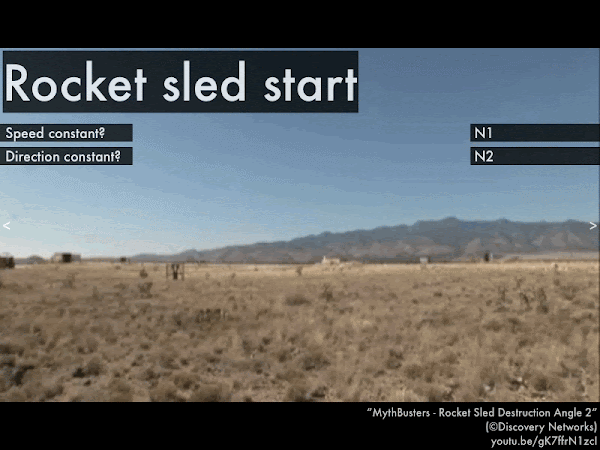(Movie link: "MythBusters--Rocket Sled Destruction Angle 2.")
For this rocket sled, Newton's __________ law applies to the motion of this object, and the forces acting on the object add up to a __________ net force.
(A) first; zero.
(B) second; non-zero.
(C) (Unsure/lost/guessing/help!)(Movie link: "F-35B Vertical Landing Highlights w/ test pilot commentary.")
While the F-35B is descending at a steady rate (before it touches the ground), Newton's __________ law applies to the motion of this object, and the forces acting on the object add up to a __________ net force.
(A) first; zero.
(B) second; non-zero.
(C) (Unsure/lost/guessing/help!)For this car with a steady speedometer reading (and assuming the shaking is due to the person holding camera, and not from moving the car itself), Newton's __________ law applies to the motion of this object, and the forces acting on the object add up to a __________ net force.
(A) first; zero.
(B) second; non-zero.
(C) (Unsure/lost/guessing/help!)(Movie link: "Spinning Swing ride at Lightwater Valley Theme Park.")
For a person in the swinging chair ride moving along a circular trajectory at a constant speed, Newton's __________ law applies to the motion of this object, and the forces acting on the object add up to a __________ net force.
(A) first; zero.
(B) second; non-zero.
(C) (Unsure/lost/guessing/help!)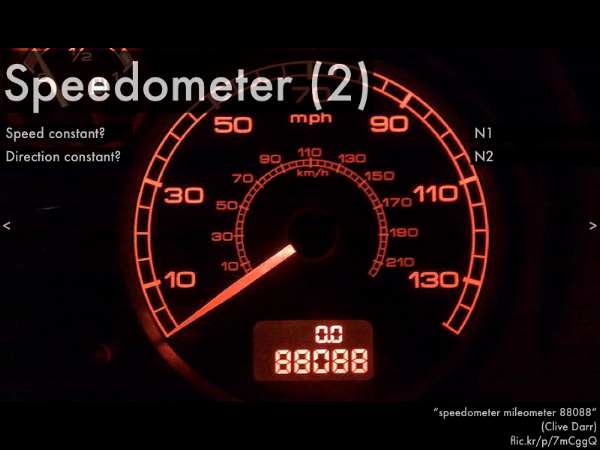For this car with a zero speedometer reading, Newton's __________ law applies to the motion of this object, and the forces acting on the object add up to a __________ net force.
(A) first; zero.
(B) second; non-zero.
(C) (Unsure/lost/guessing/help!)Now let's approach Newton's first law and second law by considering the net force on an object.Note that this net force flowchart is simpler than the motion flowchart--there is only one question that needs to be answered: Is the net force zero? If not, then Newton's second law applies. If yes, the result of adding all forces acting on an object is zero, then Newton's first law applies. Let's see how this logic flow works by looking at the forces acting on different objects.For only one force, Newton's __________ law applies to the motion of this object, and the forces acting on the object add up to a __________ net force.
(A) first; zero.
(B) second; non-zero.
(C) (Unsure/lost/guessing/help!)For two (opposite, equal magnitude) forces, Newton's __________ law applies to the motion of this object, and the forces acting on the object add up to a __________ net force.
(A) first; zero.
(B) second; non-zero.
(C) (Unsure/lost/guessing/help!)(This photo was featured on Gizmodo in 2013!)
For two (opposite, unequal magnitude) forces, Newton's __________ law applies to the motion of this object, and the forces acting on the object add up to a __________ net force.
(A) first; zero.
(B) second; non-zero.
(C) (Unsure/lost/guessing/help!)For two (diagonal, equal magnitude) forces, Newton's __________ law applies to the motion of this object, and the forces acting on the object add up to a __________ net force.
(A) first; zero.
(B) second; non-zero.
(C) (Unsure/lost/guessing/help!)

(Later, we'll consider cases where there could be three or more forces acting on an object.)Now that we know which Newton's law applies given the motion of an object, and which Newton's law applies given the net force acting on an object, let's use Newton's laws to relate the motion of an object with the net force.Newton's second law is the case where the motion of an object (whether speed, direction, or both speed and direction) changes, which means that the acceleration is non-zero. Also the net force for a Newton's second law case must also be non-zero. Since mass is non-zero, then both sides of the equation are non-zero--there must be a net force, and motion must change (speed, direction, or both speed and direction changing).What effect does mass have in Newton's second law, for accelerating objects? (Movie link: "110725-1240641-r.") The fans attached to each cart provides the same amount of force to propel it from rest.
Assuming that the drag and friction forces on each cart are also the same, the __________ cart has a greater net force exerted on it, such that it has a greater acceleration.
(A) less massive.
(B) more massive.
(C) (There is a tie.)
(D) (Unsure/lost/guessing/help!)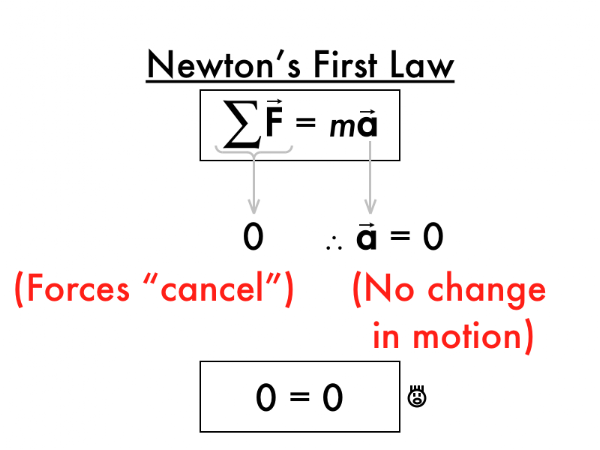Newton's first law is the case where the motion of an object is constant (both speed and direction constant), which means that the acceleration is zero. Also the net force for a Newton's first law case must be zero. Since mass is non-zero, then both sides of the equation must be zero--if there is no net force, then the motion must be constant (in both speed and direction).

Looking ahead, how many Newton's laws are there? (Three.) But there are only two ways to classify motion--constant, or changing, corresponding to Newtons first law, or second law. Or only two ways to classify net force--zero, or non-zero, corresponding to Newton's first law, or second law.

So if there are only two types of motion, and two types of net forces, what's up with Newton's third law? As it turns out, Newton's third law has nothing to do with motion or net force, but something else entirely, something much more universal and encompassing than considering a particular type of motion or net force...